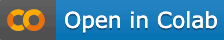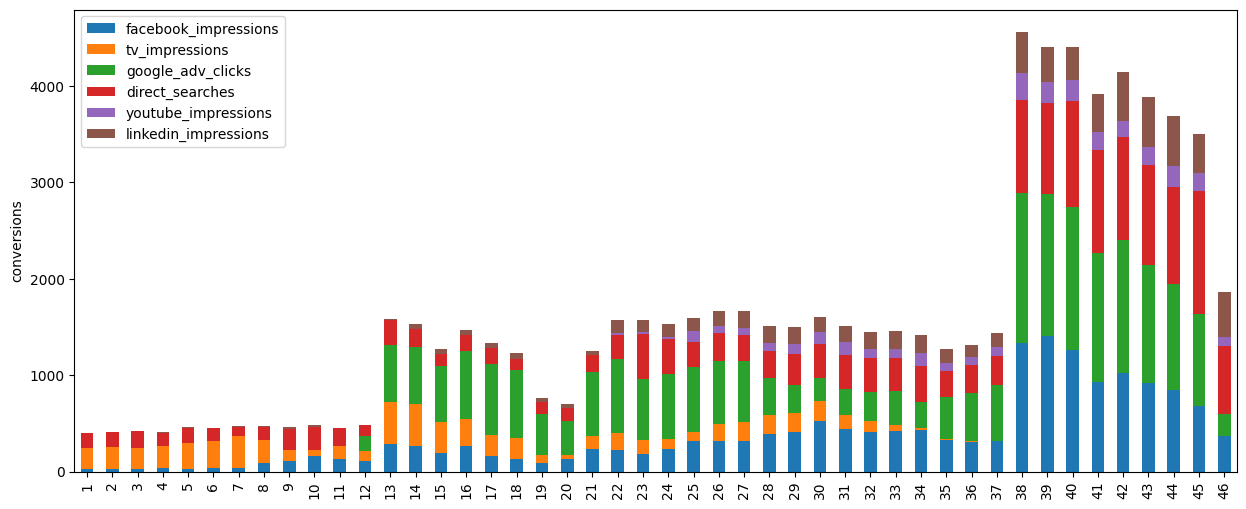### Import libraries​

``from ChannelAttributionPro import *``

``password="mypassword"``

``Data = pd.read_csv("https://app.channelattribution.net/data/Data_ata.csv")print(Data.to_string())``

### Define list of channels you are interested in performing attribution​

``channels=["facebook_impressions","tv_impressions","google_adv_clicks",          "direct_searches","youtube_impressions","linkedin_impressions"]``

### Define the global conversion rate for the use case or set it to None if not known​

``glob_conv_rate=None``

### Define the conversion rates, one for each channel if known, or set it to None otherwise​

``L_conv_rate=None``

### Define dictionary of weights (between 0 and 1), one for each channel (suggestion: use weight=1 for clicks)​

``L_weight=dict()L_weight["facebook_impressions"]=0.01L_weight["tv_impressions"]=0.01L_weight["google_adv_clicks"]=1L_weight["direct_searches"]=1L_weight["youtube_impressions"]=0.01L_weight["linkedin_impressions"]=0.01``

### Define the how many units of time are long the customer journeys on average for the use case considered​

``mean_cj_length=1``

### Define the adstock rates (between 0 and 1), one for each channels​

``L_ads_rate=dict()L_ads_rate["facebook_impressions"]=0L_ads_rate["tv_impressions"]=0.40L_ads_rate["google_adv_clicks"]=0L_ads_rate["direct_searches"]=0L_ads_rate["youtube_impressions"]=0L_ads_rate["linkedin_impressions"]=0``

### Run the model​

#### we set nsim=1 for speed reasons but at least nsim=10 is suggested​

``res=hta_model(Data, var_time="week", var_conv="conversions", channels=channels,               var_value=None, glob_conv_rate=glob_conv_rate,               L_conv_rate=L_conv_rate, L_weight=L_weight, mean_cj_length=mean_cj_length,               L_ads_rate=L_ads_rate, verbose=True, nsim=1, password=password)print(res.round(2).to_string())``

### Plot results​

``import matplotlib.pyplot as plt fig,ax = plt.subplots(figsize=(15,6))#res.set_index('week',inplace=True)res.index=range(1,len(res)+1)del res['week']res.plot(kind='bar',ax=ax,stacked=True)plt.ylabel('conversions')plt.show()``